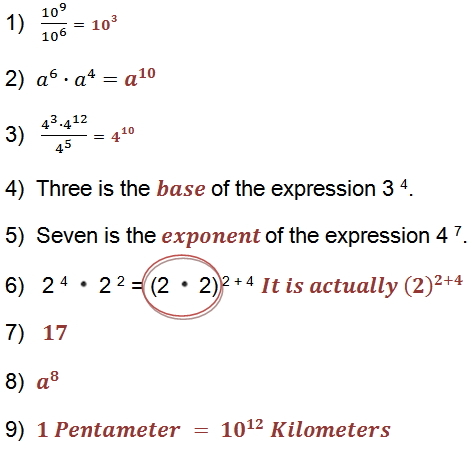Lesson - Rules of Exponents

Rules of Exponents

You can expand expressions to find their product. The following equation suggests a rule for multiplying powers with the same base when the exponents are integers.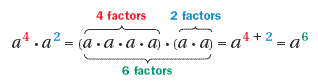Product of Powers Property
To multiply powers with the same base, add their exponents.

Rule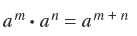Example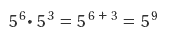Using the Product of Powers Property

Example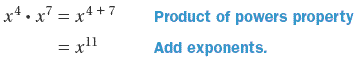Using the Product of Powers Property

Example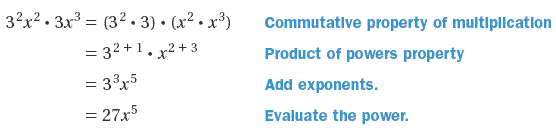The following equation suggests a rule for dividing powers with the same base when the exponents are integers.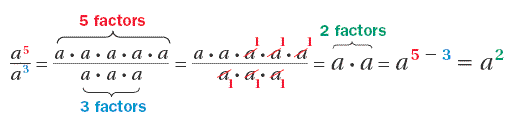Quotient of Powers Property

To divide two powers with the same base, subtract the exponent of the denominator from the exponent of the numerator.

Rule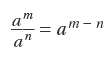Example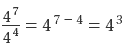Using the Quotient of Powers Property

Example 1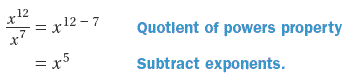Example 2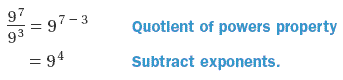1)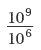2)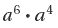Simplifying Fractions with Powers

Example 1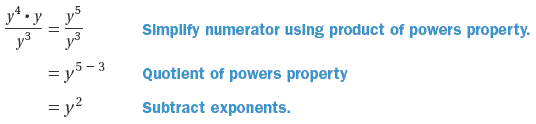Example 2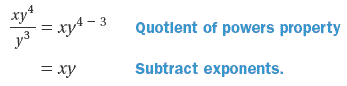3)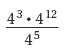Complete each statement.

4)  Three is the _____________ of the expression 3 4.

5)  Seven is the _____________ of the expression 4 7.

6)  Find and correct the error.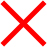4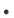2 2 = (22) 2 + 4

=  4 6

7)   What is the value of 3 23 2  -  4 3 ?

8)  What is another way to write the expression below?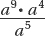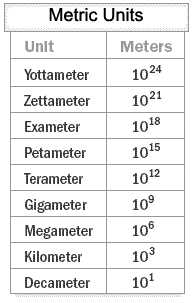9)  The table shows the number of meters in some metric measures. They are written as powers of ten.

How many kilometers are in a pentameter?